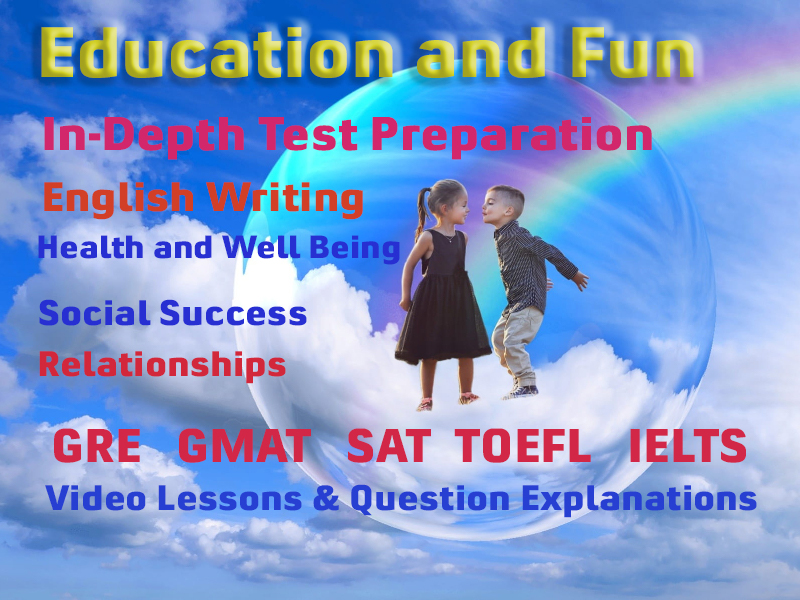# Challenging Arithmetic

Quantitative section of the test commonly known as mathematics is found in almost all admission and employment tests. The math skills required for quantitative section of any standardized test are of a basic level that should be within the reach of a high school student. You don't need to learn up lots of new formulae but you will need to sharpen up your thinking skills.

## How to Deal

Here, you'll find lessons from very beginning to the advanced level. Learn each lesson thoroughly. Keep in mind that the syllabus and course outlines on for the exam consists of topics normally covered in the 6th or 7th grads classes during your studies in a higher secondary schools. Many of you will be familiar to most of the topics but it is better to go through the whole module as you might find many new tricks.

## Use of Calculator

In some tests like SAT Reasoning, Use of calculator is permitted but we suggest you not to use the calculator during the study through this module. You must not use calculator.

Basic arithmetic is the root of the exam you are going to take. Don't ignore it even if you are already know many arithmetic operations.

Challenging Arithmetic includes the topics:

• Number System
• Addition, Subtraction, Multiplication, and Division
• LCM and HCF
• Exponents and Roots of numbers

### Test Prep Lessons With Video Lessons and Explained MCQ

Large number of solved practice MCQ with explanations. Video Lessons and 10 Fully explained Grand/Full Tests.

### What are Arithmetic Questions

The Arithmetic test (22 questions) measures your skills in three primary categories:

• Operations with whole numbers and fractions. This includes addition, subtraction, multiplication, division and recognizing equivalent fractions and mixed numbers.
• Operations with decimals and percents. This category includes addition, subtraction, multiplication, and division as well as percent problems, decimal recognition, fraction and percent equivalences, and estimation problems.
• Applications and problem-solving. Questions include rate, percent, and measurement problems, geometry problems, and distribution of a quantity into its fractional parts.

### More Educational and Fun StuffMore In-depth knowledge about what you need. Detailed about test preparation, English Writing, TOEFL, and IELTS.